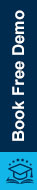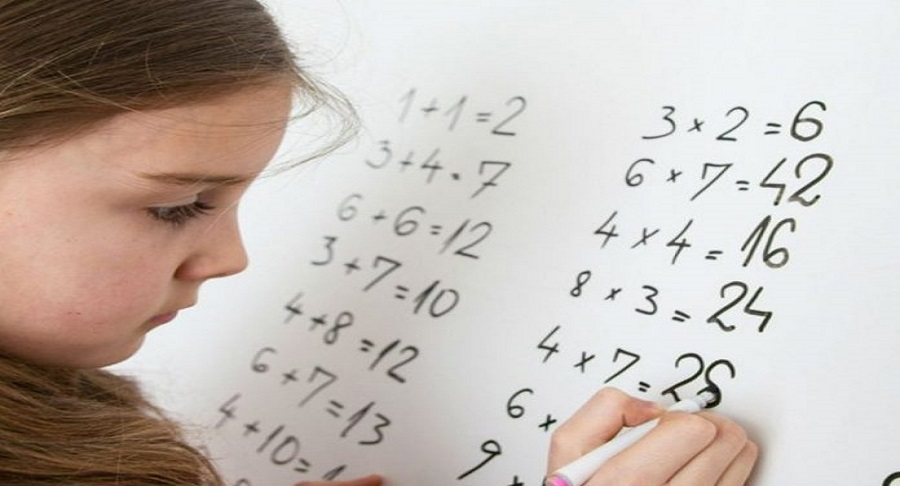# IBGA’s Delivery mechanism to teach mathematics effectively05/10/2022

All the mathematics teachers must remember that there must be IB/IGCSE academic standards that should be maintained in the classrooms.

For mathematics, the first academic standard is conceptual & problem-solving skills, which means whether the child has got an idea about the basic concepts related to the topic along with the child’s ability to solve the problems. The second one is reasoning and proof, and in this, it is observed by the teacher that if the child is able to assign a reason for the statements he is making.

The third one is relations or connections, so when there are a number of variables in mathematics it is observed by a teacher if the child is able to set up the relation between variables or can he get a formula that connects these variables, this is what we mean understanding by relations.

The fourth one if communication and this is important in mathematics, the statements written or the ideas expressed by students in mathematics must be meaningful. The last one in mathematics is data presentation, which means that it is important if the child is able to draw graphs. These are all the grounds where an IB math tutor in Delhi from IBGA understands if the child knows to represent the data.

21st century mathematical skills meant for learners to develop

Mathematics is a subject where we talk about honing 21st-century skills like critical thinking, collaboration, communication, and creativity. A lot of thinking is developed in mathematics and therefore it is not only the teacher who must solve the mathematical problems on the board, but it is parallel important that the students should understand the solution to the problem with help of the developed logic. This makes critical thinking important.

Collaborative work is the second part of contemporary skills. There are several factors that portray the significance of collaborative work in academia. And out of which, if there is a mathematical project for a team then the team spirit is developed among the learners sharing the same mathematical project.

It is important to communicate the ideas with the help of the construction of good sentences. This shows how good one is at diction and makes stamen in mathematics. Such learners have the ability to express new ideas in mathematics even if they are taking help from an online IB maths tutor in India from IBGA.

With the help of a skill called creativity, the learners make some contribution to the existing mathematical knowledge. This means they have a solution to the problem with a different approach.

IBGA resources of teaching mathematics and their importance

Course outline

It is important for the teachers to plan for lessons because it simply helps the teachers to set a vision and mission for their subjects (learners). This approach helps the teachers to set the targets, which is what development they want to see in their students. This development is in terms of the knowledge that learners have gained and they could apply it with analysis. The plan for lessons contains instructional objectives.

By an IB/IGCSE math tutor the course outline measure includes the topic introduction, warm-up exercises, and assessment of the previous mathematical knowledge, it can be motivating and preparing for the particular topic.

Activities- Projects

Mathematics is all about conducting activities, which is for the learning purpose. Activity oriented method has to be seriously employed in mathematics classrooms. When an IB math tutor feels that he/she has reached the logical conclusion to some of the learning outcomes then there must be some kind of assessment, just to ascertain the fact that learners have learned something.

It is very important for an IGCSE/IB mathematics tutor to perfectly place the assessments between the activities. The teacher can assign some homework (practice exercise), which is important for reinforcement, to strengthen the ideas that have been learned. So the given activity is used for the learning purpose, and if the teacher wants to make the learner understand an application of what has been learned, the teacher must give them a project.

The project given by the teacher is an application, it is simply the work experience of what has been learned. And that is the reason why academia talks about project-based learning.

Visual learning- Flash Cards teaching method

The image printouts must be used by the teachers to use as flashcards for example showing the figures like algebraic expressions, geometrical figures, etc. Therefore, a teacher from IBGA can conduct a quiz using the flashcard approach. This approach from an IB math tutor in Gurgaon is considered effective for the middle year program (MYP) learners from IB.

Worksheets

Worksheets have got a distinct advantage as the students read, think, and write together at the same point in time. The worksheets are for the self-assessment of the learners, but assessment here is used as a learning tool. Worksheets are also helpful for the teachers to know how effectively they teach.

A teaching tool can take numerous shapes, and each teacher, parent, and kid will interpret it differently. However, the essential concept is straightforward:

The teaching resources are pieces of content created to aid learning and knowledge intake.